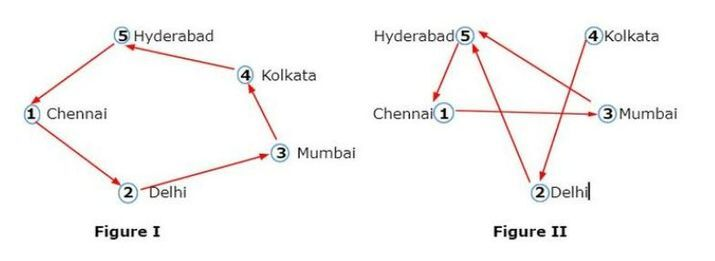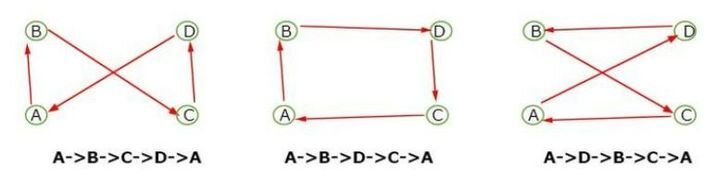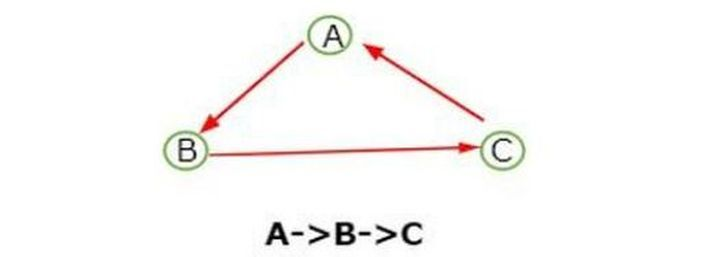# Proof that travelling salesman problem is NP Hard

The travelling salesman problem(TSP) is a solution where a salesman has to start from one place and go to all other cities just once and then come back to their own place. TSP is all about finding the minimum distance path. The polynomial-time hardness is called NP Hard which defines the property of a class of problems. The subset sum is a simple example of NP hard problem.

NP-hard − The class of problem which cannot be solved within a polynomial time is called NP-hard.

Let’s take an example of five cities to understand how salesmen travel to each city by taking the minimum distance.Which one we will prefer among these two possibilities of figure?

In these two figures, figure II represents the least/minimum distance path. In figure I, when salesmen travel from Chennai(point 1) to Hyderabad(point 5) it takes a lot of time to reach its own location and total distance covered i.e.( 1 -> 2 -> 3 -> 4 -> 5 -> 1 ) but in the case of figure II the salesman directly reach the point at 3(Mumbai) which saves the time and also cover the total minimum distance i.e. ( 1 -> 3 -> 5 -> 1 ).

Can we find the possibilities of 32 cities problem?

The possibility is given by (n-1)!/2 solution.

• In the case of 4 cities problem,

we have (4-1)!/2 = 3!/2

So there is a total of 3 possibilities for covering the distance.

Let’s look at the picture and understand the possibilities of 4 cities.• In the case of 3 cities problem, there is only 1 possibility for covering the distance.Now, let takes the problem of 41 cities where we can understand the NP-hard problem.

In the case of 41 cities, we will have 40!/2 solutions for a salesman to travel to each city.

If the salesman reaches each city and finds the best possible route it will take

• 40! / (2*106) seconds

Or 40! / (2*106*24*60*60) days

Or 40! / 365*(2*106*24*60*60) years = 6.8 * 1013 times which is more than the life of earth. Therefore, it is impossible to find all possible routes and get the optimal solution and this type of problem is called NP-hard.

## Conclusion

We explored the concept of a traveling salesman problem in NP Hard. We saw many figures which simply give the meaning of minimum distance and find the best possible route but when we come to know the NP Hard problem we observe that this type of problem cannot be solved within a polynomial time. So, this is NP Hard.

Updated on: 10-May-2023

1K+ Views